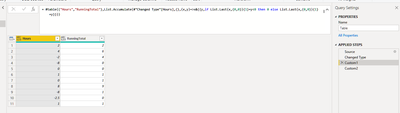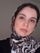cancel
Showing results for
Did you mean:Microsoft

## Running total that outputs "0" if the sum is negative, and resets running total on next row + Power

Hi All,

I am trying to create a formula that calculates the running total of column A.

However, when the running total is negative, two things should happen:

1.      the output should be 0
2.      The sum should start anew on the next row.

And most importantly... the formula cannot use column B as a reference. The formula being unable to reference itself may seem nonsensical, but the desired output is a logic puzzle of sorts. Later when using PowerPivot and power bi this concept will be important.

Is this possible with one fluid formula?

Any help is appreciated!

 Hours Wrong Running Total of [Hours] Desired Output Comments 2 2 2 4 6 6 -2 4 4 -8 -4 0 Because B5<0, the desired output should be 0 0 -4 0 New running total starting at A6 1 -3 1 0 -3 1 8 5 9 -8 -3 1 -2.5 -5.5 0 1 -4.5 1

8 REPLIES 8Super User

it would be easier to use M to resolve this.Frequent Visitor

hi.

I need the formula you wrote in this picture and I wrote that but did'nt work for me and I met with a cyclic refrence Error.:(((Microsoft

Hi Daniel,
Thank you for the response. I cannot use the M query as the main column is depending on other columns from different tables.Super User

OK, I give up. I know that technically DAX does not support recursions beyond the simple aggregator functions, yet the solution still feels to be somewhat within reach (with a calculated table variable, I am sure).  But this is not my time to solve it.Super User

I am still thinking about it. Meanwhile here is a "solution".  (It's a joke, just to be clear). zero based index added in PQ

Table Puzzle:

``````let
Source = Table.FromRows(Json.Document(Binary.Decompress(Binary.FromText("i45WMlKK1YlWMgGTuhCOrgWYMgCThkhsC2RpXSM9U5iKWAA=", BinaryEncoding.Base64), Compression.Deflate)), let _t = ((type nullable text) meta [Serialized.Text = true]) in type table [Hours = _t]),
#"Changed Type" = Table.TransformColumnTypes(Source,{{"Hours", type number}}),
in

Measure:

``````Result =
var i = max(Puzzle[Index])
return sumx(filter(all(Puzzle),Puzzle[Index]<=i),Puzzle[Hours]+if(Puzzle[Index]=3,4,0)+if(Puzzle[Index]=9,1.5,0))``````

Does it need to be a measure or can it be a calculated column?Microsoft

Both will work sirSuper User

Is it acceptable to add an index column? And this has to be DAX, not M ?Microsoft

Thank you for the response Ibendlin. Yes, it is acceptable and this had to be written in dax.